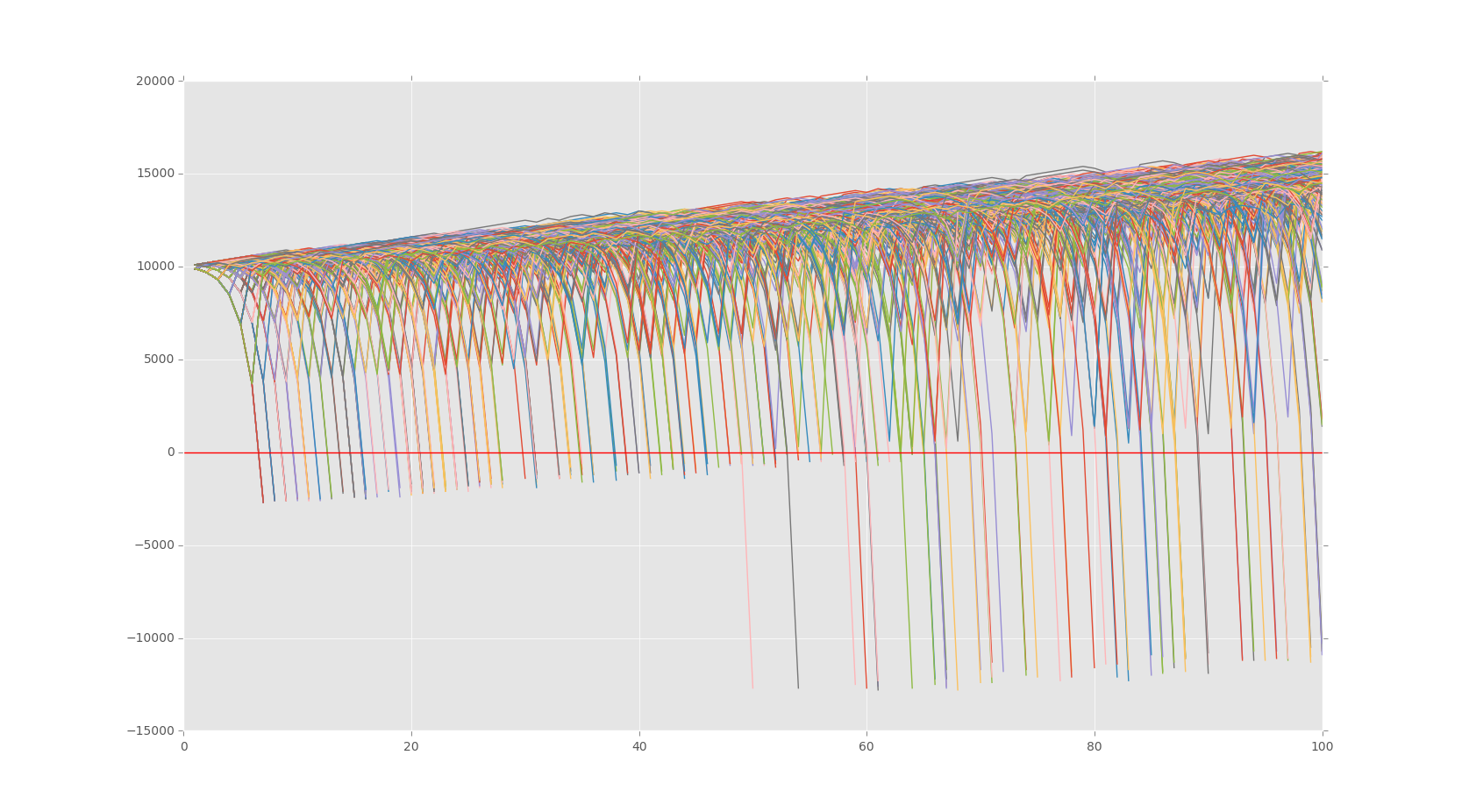## Bettor Statistics

```import random
import matplotlib
import matplotlib.pyplot as plt
import time

def rollDice():
roll = random.randint(1,100)

if roll == 100:
return False
elif roll <= 50:
return False
elif 100 > roll >= 50:
return True

def doubler_bettor(funds,initial_wager,wager_count):
global broke_count
value = funds
wager = initial_wager
wX = []
vY = []
currentWager = 1

# since we'll be betting based on previous bet outcome #
previousWager = 'win'

# since we'll be doubling #
previousWagerAmount = initial_wager

'''
immediately with these comments, and our previous discussion of how previous outcomes
do not affect future outcome possibilities, you should realize that this betting method
offers nothing more than a quicker realization of losses or gains.

Another way to visualize this quicker realization is actually an increase in risk.
This bettor will experience extremely unpredictable volatility most likely.
'''

while currentWager <= wager_count:
if previousWager == 'win':
##print 'we won the last wager, yay!'
if rollDice():
value += wager
##print value
wX.append(currentWager)
vY.append(value)
else:
value -= wager
previousWager = 'loss'
##print value
previousWagerAmount = wager
wX.append(currentWager)
vY.append(value)
if value < 0:
##print 'went broke after',currentWager,'bets'
broke_count += 1
currentWager += 10000000000000000
elif previousWager == 'loss':
##print 'we lost the last one, so we will be super smart & double up!'
if rollDice():
wager = previousWagerAmount * 2
##print 'we won',wager
value += wager
##print value
wager = initial_wager
previousWager = 'win'
wX.append(currentWager)
vY.append(value)
else:
wager = previousWagerAmount * 2
##print 'we lost',wager
value -= wager
##print value
previousWager = 'loss'
previousWagerAmount = wager
wX.append(currentWager)
vY.append(value)
if value < 0:
##print 'went broke after',currentWager,'bets'
currentWager += 10000000000000000
broke_count += 1

currentWager += 1

##print value
plt.plot(wX,vY)

xx = 0
broke_count = 0

while xx < 1000:
doubler_bettor(10000,100,100)
xx+=1

#print 'death rate:',(broke_count/float(xx)) * 100
#print 'survival rate:',100 - ((broke_count/float(xx)) * 100)
plt.axhline(0, color = 'r')
plt.show()
```The next tutorial:• Monte Carlo Introduction

• Monte Carlo dice Function

• Creating a simple Bettor

• Plotting Results with Matpltolib

• Martingale Strategy

• Bettor Statistics
• More comparison

• Graphing Monte Carlo

• Fixing Debt Issues

• Analyzing Monte Carlo results

• Using Monte Carlo to find Best multiple

• Checking betting results

• D'Alembert Strategy

• 50/50 Odds

• Analysis of D'Alembert

• Comparing Profitability

• Finding best D'Alembert Multiple

• Two dimensional charting monte carlo

• Monte Carlo Simulation and Python

• Labouchere System for Gambling Tested